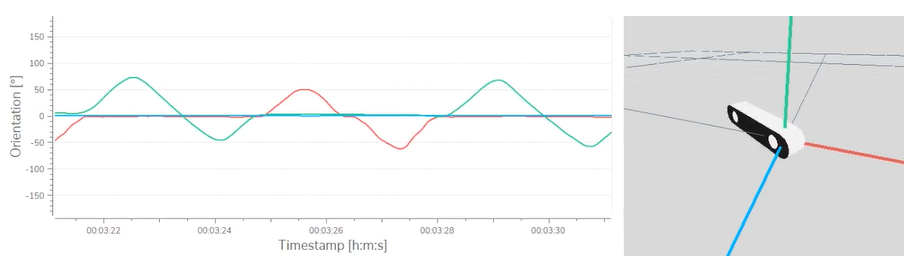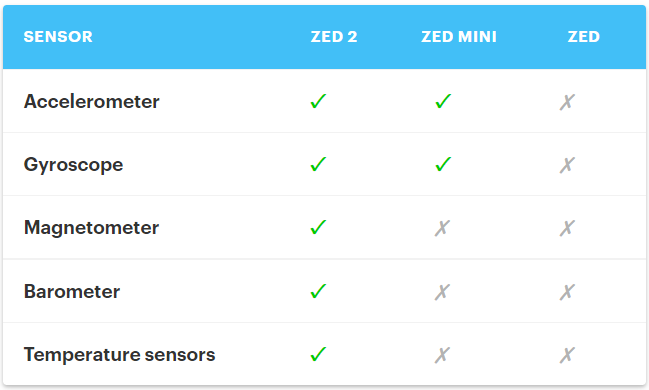# ZED 景深攝影機範例#7/8 – Using sensors

1. Hello ZED: 入門範例，說明如何連接 ZED 攝影機，並於終端機中顯示裝置序列編號。
2. Image Capture: 開啟 ZED 攝影機，取得影像，並於終端機中顯示時間戳記、影像尺寸。
3. Depth Perception: 取得畫面的深度資料與點雲，並於終端機中顯示指定點的距離。
4. Camera Tracking: 啟用位置追蹤，可即時更新攝影機的位置與指向。
5. Spatial Mapping: 啟用地圖繪製，可擷取環境的網格或融合點雲。
6. 3D Object Detection: 偵測畫面中的物體，並進行 3D 定位 （只適用於 ZED 2 機型）。
7. Using Sensors: 取得攝影機的 IMU、氣壓計與磁力感測器資料。
8. Body Tracking: 追蹤人體骨架

# 範例07 – 取得感測器資料## 前置作業

• 下載最新版的 ZED SDK請點我
• 下載 Image Capture 範例程式，提供 C++, Python 與 C# 等版本
• 在 Windows 或 Linux OS上，建置 C++ 環境(請點我) 或執行 Python 範例，本系列文章將使用 Python （教學請點我

## 程式總覽

### 開啟攝影機

open the camera
``# Create a ZED camera objectzed = sl.Camera()# Set configuration parametersinit_params = sl.InitParameters()# No depth computation required hereinit_params.depth_mode = sl.DEPTH_MODE.NONE# Open the cameraerr = zed.open(init_params)if err != sl.ERROR_CODE.SUCCESS :    print(repr(err))    zed.close()    exit(1)``

### 取得感測器資料

capture sensor data
``sensors_data = sl.SensorsData()while zed.grab() == sl.ERROR_CODE_SUCCESS:    zed.get_sensors_data(sensors_data, sl.TIME_REFERENCE.CURRENT)``

### 更新感測器資料

store timestamps and check for data update
``ts_handler = TimestampHandler()if ts_handler.is_new(sensors_data.get_imu_data()):    # sensors_data.get_imu_data() contains new data``

display new data in console
``# Check if IMU data has been updatedif ts_handler.is_new(sensors_data.get_imu_data()):    quaternion = sensors_data.get_imu_data().get_pose().get_orientation().get()    print("IMU Orientation: {}".format(quaternion))    linear_acceleration = sensors_data.get_imu_data().get_linear_acceleration()    print("IMU Acceleration: {} [m/sec^2]".format(linear_acceleration))    angular_velocity = sensors_data.get_imu_data().get_angular_velocity()    print("IMU Angular Velocity: {} [deg/sec]".format(angular_velocity))# Check if Magnetometer data has been updatedif ts_handler.is_new(sensors_data.get_magnetometer_data()):    magnetic_field_calibrated = sensors_data.get_magnetometer_data().get_magnetic_field_calibrated()    print("Magnetometer Magnetic Field: {} [uT]".format(magnetic_field_calibrated))# Check if Barometer data has been updated if ts_handler.is_new(sensors_data.get_barometer_data()):    magnetic_field_calibrated = sensors_data.get_barometer_data().pressure    print("Barometer Atmospheric pressure: {} [hPa]".format(sensors_data.get_barometer_data().pressure))``

### 關閉攝影機

close the camera
``# Close the camerazed.close()``

## 下一步